CATEGORIES:

HETEROGENOUS EQUILIBRIUM

In a saturated solution of a sparingly soluble strong electrolyte, an equilibrium sets between the precipitate (solid phase) of the electrolyte and its ions in the solution:

Ag3PO4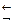3 Ag+ + PO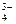This equilibrium state may be characterized by a constant called a solubility product, Ksp:

Ksp (Ag3PO4) = [Ag+]3 [PO]

When the concentration of one of the ions of an electrolyte in its saturated solution is increased (for instance, by introducing another electrolyte containing common ions), the product of the concentrations of the electrolyte ions becomes greater than Ksp. In this case the equilibrium between the solid phase and the solution shifts towards the direction of formation of a precipitate.

Consequently, the condition for formation of a precipitate is the greater value of the product of the concentrations of the ions belonging to a sparingly soluble electrolyte in comparison with its solubility product.

Conversely, if the concentration of one of the ions in a saturated solution is diminished (for example by combining it with another ion), the product of the ion concentrations will be lower than the value of Ksp, the solution will become unsaturated and the equilibrium between the solid phase and the solution shifts towards the dissolution of the precipitate.

The value of Ksp can be used to calculate the solubility of sparingly soluble electrolytes in water and in solutions containing other electrolytes (solubility can be determined as a molar concentration of a dissolved substance).

The shift of an equilibrium of a chemical reaction can be determined theoretically by calculation of an equilibrium constant of a chemical reaction:

K=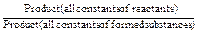If the K value exceeds 1, the equilibrium of a chemical reaction is shifted towards the forward reaction. In case of K<1 the equilibrium shifts to the direction of a backward reaction.

EXPERIMENTAL PART

1. Formation and dissolution of a precipitate

Take 3-4 drops of a solution of any calcium salt into a test tube and add a solution of ammonium oxalate until the formation of a precipitate. Dissolve the precipitate in hydrochloric acid. Compare the values of the dissociation constants of oxalic acid and the solubility product constant for calcium oxalate and make your conclusion.

2. Direction of a chemical reaction

a) Take 3-4 drops of any barium salt in a test tube and add potassium chromate until the formation of a precipitate. Divide the precipitate into two parts and add HCl or CH3COOH respectively. Notice your observations.

b) Take 3-4 drops of any copper salt in one test tube and 3-4 drops of any manganese salt in another test tube. Add sodium sulfide until metal sulfide precipitates is formed. Try to dissolve each precipitate in a diluted solution of hydrochloric acid.

Calculate equilibrium constants of the reactions of dissolution of precipitates and make your conclusion about the possibility of each reaction.

3. Heterogenous equilibrium

a) Mix 5-6 drops of silver nitrate with 2-3 drops of sodium chloride in a test tube. Observe the formation of a precipitate of AgCl. Then add 2-3 drops of potassium chromate into the same test tube. Stir the mixture and observe the change in color of the precipitate. Add 2-3 drops of sodium sulfide and write down the observations.

b) Repeat the experiment changing the order of addition of reactants. Add first NaCl, then Na2S and finally K2CrO4s.

Calculate the equilibrium constants of all the reactions and make your conclusion about the shifting of chemical equilibria.

QUESTIONS AND PROBLEMS

1. Calculate the solubility product constant of PbBr2 if the solubility of the salt is 1.32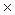10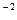mole×l-1.

2. Will a precipitate of silver sulfate be formed if a 1M solution of H2SO4 is added to an equal volume of a 0.02 M solution of AgNO3?

3. Calculate the equilibrium constant of the reaction and explain whether the precipitate of calcium oxalate can be dissolved in acetic acid.

Experiment 3

Date: 2015-01-12; view: 806

 <== previous page | next page ==> EXPERIMENTAL PART | IONIC PRODUCT OF WATER. PH. HYDROLYSIS OF SALTS
doclecture.net - lectures - 2014-2022 year. Copyright infringement or personal data (0.002 sec.)